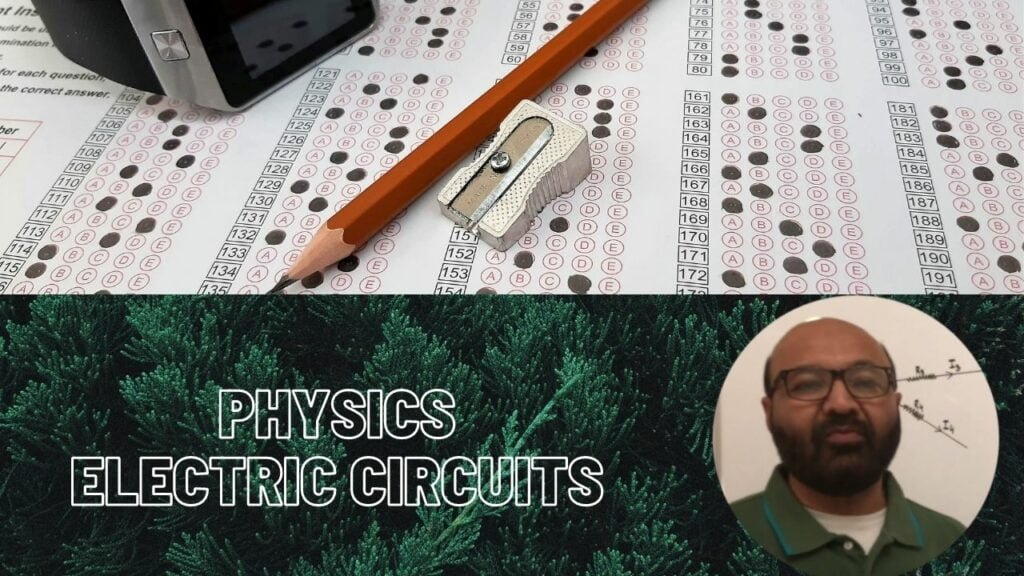Physics Lectures### Electric Circuits

One simple way of knowing electric circuits is, to recall what do you do when you enter into home? You press electric switch and instantly light bulb turn on. You press TV button and it turns on and the moment you press the button again it turns off the TV. It means that these electrical appliances are connected via some metal wires and eventually connected with a source of electricity. These metal wires provide to and fro path for flow of electric current. This path of electric current is called electric circuit.

When you disassemble any electric appliance, inside that you find tiny electrical devices connected in the circuit board. One of which is resistor that controls the amount of current flow in a circuit. Function of a resistor resembles water tap. Flow of water through the tap depends on how much tap you open. The more you open it the more it allows water to flow through it. When you close it flow of water stops. For resistors it is its material which controls the flow of electric current through it. Some materials allow high amount of current flow while others allow small amount of current flow through it.

RESISTORS IN SERIES

If in any electric circuit two or more resistors are connected end-to-end, it is called resistors in series.

The amount of current flows through a set of resistors in series will be the same at all the points in the circuit.

IR1 = IR2 = IR3 = Iab = 1mA

Total voltage in a series circuit divides among the individual resistors; so the sum of the voltage of individual resistors is equal to the total voltage supplied by the source. Thus

V1 = IR1 = 2V
V2 = IR2 = 4V
V3 = IR3 = 6V
VT = V1 + V2 + V3
VT = 2V + 4V + 6V = 12V

Where VT is the total voltage across the circuit, and V1, V2, V3 are the voltages across resistors R1, R2, R3 respectively. If I is the current passing through each resistor, then according to Ohm’s law

V = IR
V = IR1 + IR2 + IR3
V = I (R1 + R2 + R3)
Then IR = I (R1 + R2 + R3)
RT = R1 + R2 + R3
RT = 2 K? + 4 K? + 6 K? = 12 K?

Thus, in series circuit the total resistance is equal to the sum of individual resistances in the circuit.

Where are the series circuit used?

When we need the same current in the whole circuit, we use series circuit because in this type of arrangement current remains the same at all the points in the circuit. In other words, series circuits are used in situations where operation of a circuit is required to be linear. It is our daily life observation that series circuits are used for lighting. The most familiar example is string of LEDs used for decoration purposes and runway lights used for guiding pilots at the time of landing of an aeroplane.
A series circuit must be able to cope with the sum of all resistances on the circuit. Thus, the voltage must be high enough to compensate for the voltage drop from each resistor.

RESISTORS IN PARALLEL

Resistors are said to be connected parallel if two or more resistors connected side-by-side across two points ‘a’ and ‘b’ in a circuit. The current enters at the point ‘a’ has three possible paths to flow. Current splits into the amount I1 for the resistor R1, I2 for the resistor R2 and I3 for the resistor R3. Then parallel circuits are classed as current dividers. The amount of current enters at point ‘a’ must be equal to the sum of all currents at point ‘b’ reaching through resistors R1, R2 and R3 i.e.

I = I1 + I2 + I3

The resistors in parallel have a common voltage across them; then voltage across resistor R1 is equal to the voltage across resistor R2, equal to the voltage across resistor R3 and equals to the source voltage.

VR1 = VR2 = VR3 = Vab
The current across the resistors R1, R2, and R3 are:
I1 = V/ R1 I2 = V/ R2 I3 = V/ R3

The total current I = I1 + I2 + I3

I = V/ R1 + V/ R2 + V/ R3
I = V (1/ R1 + 1/ R2 + 1/ R3)
If I = V/R V/R = V (1/ R1 + 1/ R2 + 1/ R3)

The equivalent resistance is 1/R = 1/ R1 + 1/ R2 + 1/ R3
For resistors in parallel connection, the equivalent resistance is always less than the smallest resistor in the circuit.

Where are the parallel circuits used?

A parallel circuit is used when the current through several components need to be independent of each other. The total available battery current is increased when cells are arranged in parallel. Your car battery is an example of such kind.

Tags :